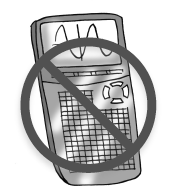### Home > INT1 > Chapter Ch10 > Lesson 10.1.2 > Problem10-26

10-26.A sample of 23 newborns at the Dallas University Health Center had the following lengths in centimeters. The measurements have been sorted from lowest to highest. Homework Help ✎

46.4, 46.9, 47.7, 48.1, 48.5, 48.5, 48.8, 49.0, 49.3, 50.0, 50.1, 50.4, 50.6, 51.1, 51.4, 51.8, 52.4, 52.5, 53.2, 53.8, 54.4, 55.1, 55.9

Find the five-number summary of the lengths of the newborns. For help, see the Math Notes box in this lesson.

To find the minimum and the maximum, find smallest and largest numbers in the list. Because the list is ordered from least to greatest, the smallest will be first and the largest will be last.

The median is the number in the middle, so in a data set with 23 numbers, the median would be the 12th number. It has been underlined for you.

The first quartile is the median of the first half of the data. In this case, it would be the 6th number. The third quartile is the median of the second half of the data. In this case it would be the 18th number. These numbers have been underlined for you.

Minimum: 46.4, First Quartile:48.5, Median: 50.4, Third Quartile: 52.5, Maximum: 55.9